# Detailed Procedure for Concrete Mix Design

Concrete is a composite material that essentially consists of water, a binding medium (cement) embedded with Fine-Aggregate (typically sand) and Coarse Aggregate (typically gravel) with or without chemical and mineral admixture.

Per capita Consumption of concrete is only next to water , concrete is a critical construction material and it costs around 8- 12% of project cost, understanding concrete design can improve  construction quality and can help save cost of construction.

At site level “concrete” is left to quality control engineers, hope this blog help the execution engineer to understand it better and implement the learning in day to day concrete activities(production, placing, finishing)

## Constituents of Concrete:

Constituents of concrete are basically mixture of aggregate and paste

• Paste 30 to 40% – Portland cement 7% to 15% by Vol & Water 14% to 21% by Vol.

Note: Higher the concrete grade, paste volume will be higher

• Aggregates 60% to 70% – Coarse aggregates & Fine aggregates.

Note:

1. Higher the concrete grade, aggregate volume will be lower
2. CA & FA proportion is generally 60:40, Finer the sand its content would be lower, higher in case of coarser fine aggregate.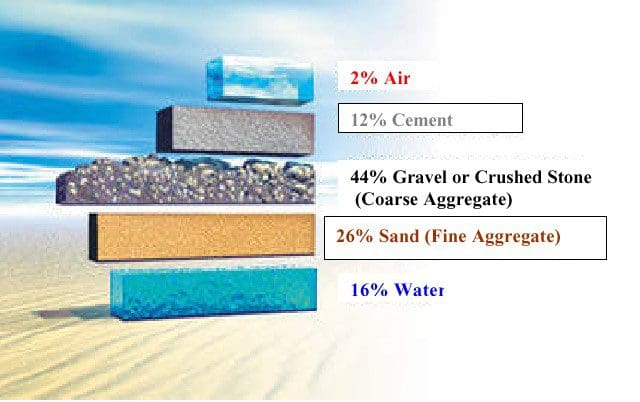Understanding basic concrete material individually would help us design a value engineered concrete mix design.

The selection of concrete proportion involves a balance between economy and basic requirement of workability, setting time and strength ,for a particular application.

Workability: The property of the concrete that determines its capacity to be placed and consolidated properly and be finished without harmful segregation. It is the relative mobility of the concrete mixture, and measured in terms of the slump; the greater the slump value the more mobile the mixture.

Setting Time: Setting time determine the time before which concrete should be placed (Initial Setting Time) to its final position (finished).It is determined using a penetrometer. Generally ranges from 01 hr to 04 hrs. (with chemical admixture).

Strength: The basic criteria which define grade on a concrete, basically capacity of the concrete to resist compression stresses at the age of 28 days.

## Background Data of Concrete Ingredients

Once the source of individual concrete ingredients are approved, the following information for available materials will be useful in designing a Concrete mix

• Sieve analyses of fine and coarse aggregates.
• Specific gravities and absorption of aggregates.
• Mixing-water requirements of concrete developed from experience with available aggregates.
• Relationship between strength and water-cement ratio.
• Specific gravity of Portland cement and other cementitious materials(flyash, ggbfs etc), if used.
• Optimum combination of coarse aggregates to meet the maximum density grading for mass concrete.

Other data which can be useful are silt content (Fine Aggregate), Flakiness and Elongation Index (Coarse Aggregate).

## Basic functions of Concrete Ingredients

### 1. Cement

Dry powder of very fine particles forms a paste when mixed with water enter into chemical reaction-Hydration forming glue like product, cement paste coats all the aggregates together finally hardens and forms a solid mass.

### 2. Water

Needed for two basic purposes, primarily for chemical reaction with cement and secondly to achieve required workability. Only 1/3 of the water is needed for chemical reaction extra water remains in pores and results in porosity. Good for preventing plastic shrinkage cracking and workability bad for permeability, strength, durability.

Water is necessarily evil in concrete, use miserly.

### 3. Aggregates

Aggregates are inexpensive fillers, provide volume stability and abrasion resistance, reduce volume changes and does not enter into complex chemical reactions with water (inert) ,it has been customary, therefore, to treat it as an inert filler in concrete.

However, due to increasing awareness of the role played by aggregates in determining many important properties of concrete, the traditional view of the aggregate as inert filler is being seriously questioned.

It is inappropriate to treat the aggregate with any less respect than cement as it occupies 60 – 70% Volume in a concrete formulation.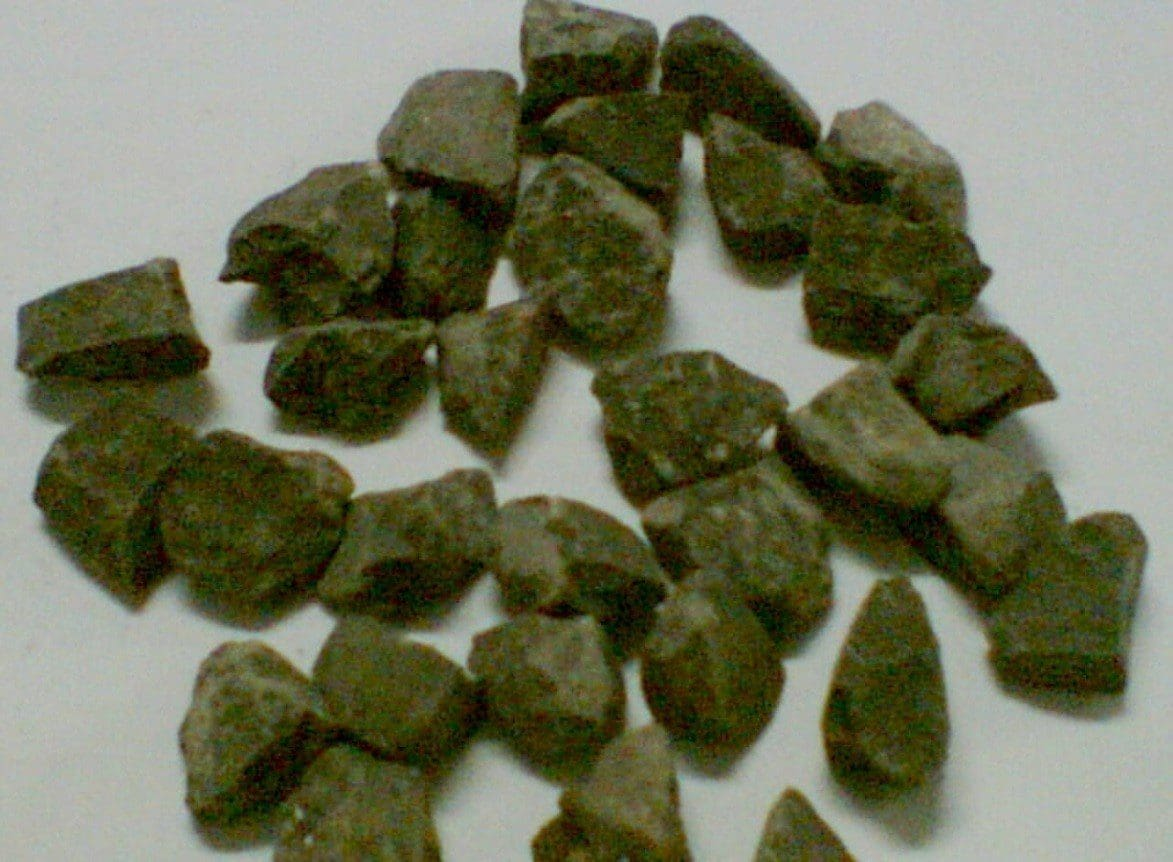Ideal Aggregate: Neither Elongated Nor Flaky

## Design Stipulations Required for Initiating a Concrete Mix Design

• Characteristic Compressive Strength

(basic mix design criteria, required to ascertain Target mean strength)

• Maximum size of Aggregate

(Governs water and cement content)

• Degree of workability

(basic placement requirement, governs water content)

• Degree of quality control

(Assumption of standard deviation, depending upon site quality control)

• Type of Exposure

(To fix minimum cement content ,maximum water – cement ratio and minimum grade of concrete)

## Test Data Required for Individual Materials (Physically characterized)

Cement

Type & Grade ( w/c ratio for target mean strength)

Specific Gravity (calculation of various ingredients)

Aggregate

Specific gravity (calculation of various ingredients)

Sieve Analysis (proportioning of fine & coarse aggregate)

## Steps of Concrete Mix Design

### Step-1: Target Mean Strength

In order that not more than the specified proportion of test results are likely to fall below the characteristic strength.

f t.m.s = f ck + t X s

f t.m.s = target mean strength at 28 days.

f ck = characteristic compressive strength at 28 days.

t = a statistic, depending upon the accepted proportion of low results = 1.65 i.e 1 in 20 failure

s = standard deviation (as per table 01)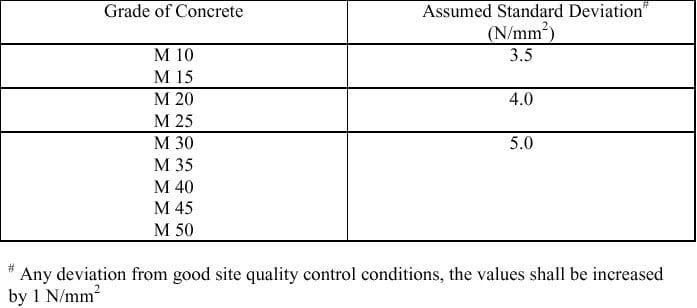Table-01

Concrete Mix design illustration for M25:

f t.m.s = f ck + t X s = 25 + 1.65 X 4 = 31.6 MPa

For M25 grade concrete the target mean strength = 31.6 MPa

In control laboratory condition we should be targeting a strength of 31.6MPa so that the characteristic strength at site should not fall below 25MPa for M25 grade concrete.

### Step-02: Selection of Water Cement Ratio

• Different cements and aggregates of different maximum size ,grading surface texture, shape and other characteristic may produce concretes of different compressive strength for the same water cement ratio
• The relationship between strength and water cement ratio should be preferably established for the materials actually to be used.
• In the absence of such data, the preliminary water- cement ratio corresponding to the target strength at 28 days may be selected from the relation shown in fig 01, alternatively by fig 02(A,B.. are compressive strength of cement being used at 28days)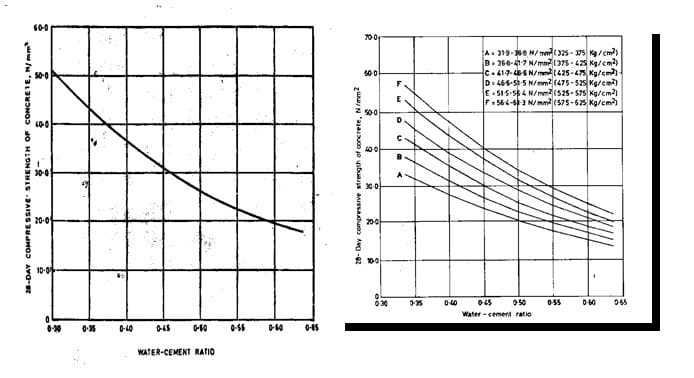Table-02

For a TMS OF 31.6 MPa the w/c ratio to be selected is 0.53

w/c =0.53

### Step-03: Estimation of Water Content and Fine to total Aggregate Ratio

Approximate sand and water content per cubic meter of concrete for grades upto M 35(refer Table 03)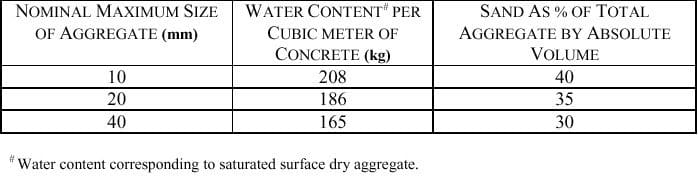Table-03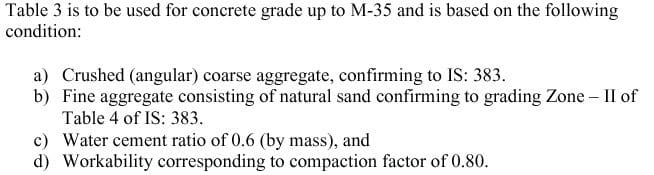Table-04

Considering Maximum aggregate size of 20 mm , the water content = 186 Kg(as per Table 03) and 35 % sand (as % of total aggregate by absolute volume)

If sand is 35% then coarse aggregate would be 65% of total aggregate volume

#### Adjustments of values in water content and Sand Percentage for Other Considerations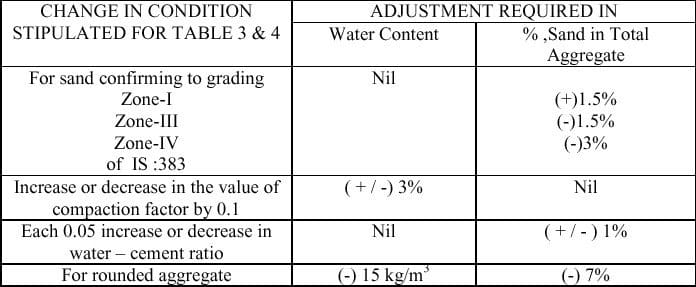Table-05

Any deviation of aggregate from the consideration of Table 03 & 04 ,table 05 adjustments to be incorporated.

i.e if sand is of zone – I ,then sand content would be 35+1.5 = 36.5%,no adjustment in water content selected in Step 3.

If workability need to be higher by compaction factor of 0.1 then water content to be increased by +3%

= 186 +0.03*186 = 191.6 kg.

Do adjustment as per available sand and required workability,if illustrated condition differ at your end.

### Step-04: Calculation of Cement Content

Water – cement ratio arrived at step-02

Water content arrived at step – 03

Cement Content = Water content / water-cement ratio

Arrived cement content and water – cement needs to be checked with Table-06 (Ref : IS 456,exposure conditions)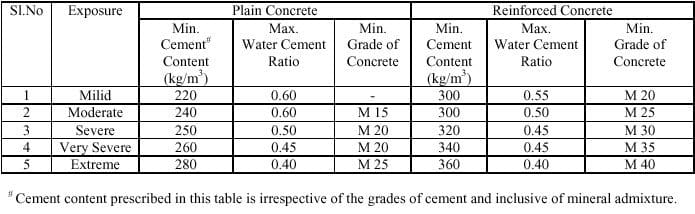Table-06 Exposure Conditions

Water – cement ratio arrived at step-02 = 0.52

Water content arrived at step – 03 = 186

Cement Content = Water content / water-cement ratio = 186/0.52 = 357.69 say 358 kg

Note : If exposure condition is Mild then Table 06 criteria is satisfied

i.e Minimum cement should be 300 ,we got 358 and w/c should be max 0.55 ,we got 0.52

ENVIRONMENTAL EXPOSURE CONDITIONS, Table – 07 (defined as per IS 456:2000)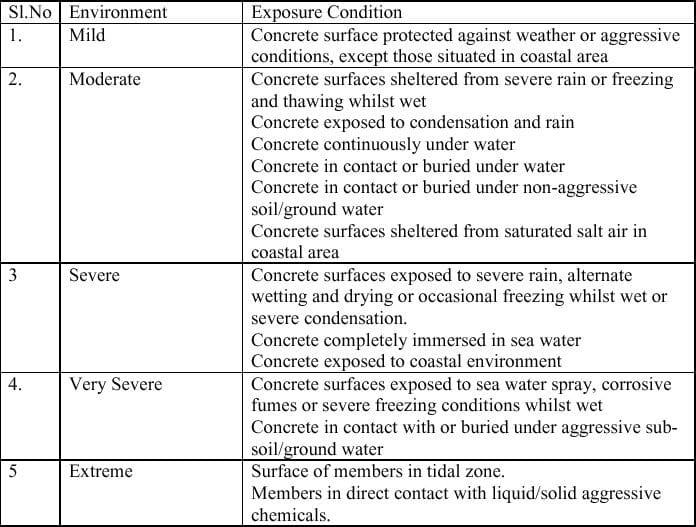Table-07 : Environmental Exposure Conditions

### Step-05: Calculation of Aggregate Content

The total aggregate content per unit volume of concrete may be calculated from the following  equations

V = [ W + C/SC + 1/p * fa /Sfa] x 1/1000

&

V = [ W + C/SC + 1/1-p * ca /Sca] x 1/1000

Where

V = absolute volume of fresh concrete, which is equal to gross volume (m3) minus the volume of assumed entrapped air.

W = mass of water (kg) per m3 of concrete [arrived in step -03 ]

C = mass of cement (kg) per m3 of concrete [arrived in step -04 ]

p = ratio of fine aggregate to total aggregate by absolute volume [arrived in step -03 ]

fa,ca =total mass of fine aggregate and coarse aggregate (kg) per m3 of concrete respectively.

Sfa,Sca = specific gravities of saturated surface dry fine aggregate and coarse aggregate respectively. [test data of materials] i.e. Sfa =2.6 ,Sca =2.8 (assuming)

S= specific gravities of cement , i.e.=3.15

Working illustration

Calculation for fine aggregate (sand)

V = [ W + C/SC + 1/p * fa /Sfa] x 1/1000

1 = [186 + 358/3.15 + 1/0.35 * fa /2.6]x1/1000

1000 = 186 + 113.65 + fa/0.91

fa/0.91 =1000-186-113.65

fa=0.91* 700.35 =637 kg

Calculation for coarse aggregate

V = [ W + C/SC + 1/1-p * Ca /Sca] x 1/1000

1 = [186 + 358/3.15 + 1/0.65 * Ca /2.8]x1/1000

1000 =186 +113.65 + Ca/1.82

Ca/1.82 =1000-186-113.65

Ca =1.82*700.35 =1275 kg

Note :Coarse aggregate of different sizes (10mm & 20mm) can be combined in suitable proportions (50:50,40:60 etc) for better results.

Arrived initial trial mix proportion for M25: